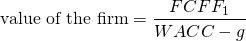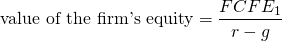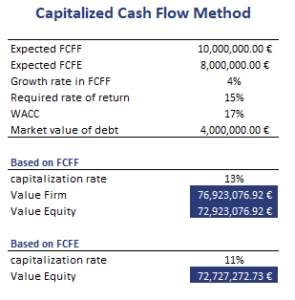# Capitalized Cash Flow Method

The Capitalized Cash Flow Method (CMM) is a method used to value private companies. It also known as the capitalized income valuation method or capitalization of earnings method. Under this method, a single value of economic benefit is capitalized at a capitalization ratio to arrive at the firm’s value. The capitalization rate equals the required rate of return minus the company’s expected growth rate. The model is commonly used to value small private companies.

### Capitalized cash flow valuation formula

As we mentioned in the introduction, the capitalized cash flow method values a company as a growing perpetuity. Thus, the approach assumes a constant growth rate. The model is very similar to a single-stage dividend discount model. If, however, the growth rate is not expected to be constant, a different approach should be used.

The formula for the capitalized cash flow method equalswhere FCFF is the expected Free Cash Flow to the Firm, WACC is the Weighted Average Cost of Capital, and g is the constant growth rate.

To determine the value of the equity, we have to subtract the market value of the firm’s debt. Alternatively, we can calculate the value of the equity directly by using the nominator the Free Cash Flow to Equity (FCFE) instead of the FCFF. If we decide to calculate the value of equity this way, we have to use the required return on equity instead of the WACC.We can also use the above formulae to back out the required return or growth rate.

## Capitalized cash flow approach example

Next, let’s consider a numerical example to illustrate how we can use the above formulas to calculate the value of a private company. The approach most useful when we do not have comparable companies, projections are uncertain, or when stable growth is a reasonable assumption. The table below illustrates the necessary calculations. We apply both the method to calculate the firm’s value as well as the method the calculate the firm’s equity.